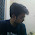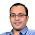### Empty Bucket

Source: Tejas Hiremani (EE, IITB) asked this question. I had read it before in Peter Winkler's puzzle book and Carnegie Mellon's Puzzle Toad site. Nice problem.

Problem:
Given 3 buckets containing x,y,z litres of water. Assume buckets have "large" size. x, y, z are integers. You can move water from one bucket to another only if the bucket to which water is being transferred doubles the amount of water it has. So, all moves have to of the form:
If water is being moved from bucket containing x litres to bucket containing y litres. After the operation, the bucket containing y litres has 2y litres and the first bucket has x-y litres. Show that you can always empty one of the buckets. :)

Update (18/12/09):
Solution:
One solution posted by Aaditya Ramdas (CSE, IITB alumnus - Tower Research Analyst) in comments. A different solution posted by me in comments.

1.ok this is a short solution coz of lack of time, im sure u can figure the details out :P E for even, O for odd.

1. E,E,E => divide by 2 and solve smaller case.
2. E,O,O => make it EEE with any move with OO
3. O,O,O => make it OEE
4. Lets solve OEE :D

See first make one, say the middle bucket, a multiple of 4 (this ull figure out why later, but its trivial to figure this out) with a move between EE.

So you have OE1E2 with E1 as mult of 4. Now make moves between first 2 buckets only. This is a finite state machine or whtever :P (you can only move from larger L to smaller S, so no move will ever empty the buckets - it will be 2S, L-S). You'll get back to OE1E2 eventually. The step JUST before that has to be O+(E1/2), E1/2, E2.

So now you're at OEE with higher O. Keep repeating this process. If the O gets higher and higher, eventually one of the Es must become empty.

2.I think that is correct!! I was expecting a better explained and more creative solution.. :)

BTW, I could have figured the details out even without your solution.. I know the solution :P

3.A different approach to the problem.

In Ramdas's solution, he is trying to increase the content of one bucket until one of the bucket becomes empty.

In this solution, we will prove that we can always decrease the minimum of the three amounts of water in the containers. If the minimum becomes zero, stop, else relabel the buckets and start the following loop again.

Label the buckets A, B, C containing a, b, c litres of water such that 0 < a <= b <= c

Let b = qa+r
Write q (binary rep) as q = q_0 + q_1*2 + q_2*2^2 ... q_n*2^n where q_n =1

Do n+1 moves, numbered 0, 1.. n as follows. In move i, if q_i=1, we pour from B into A. Else, we pour C into A. Since A's content is doubled in every move, A's content is 2^i*a after i moves. B would have poured qa litres. So, amount present in B now is b-qa=r<a. We just need to prove that there always exists a move from C to A. Note that total amount poured from C to A is less than 2^n*a <= qa <= b <= c. So, there is always enough water in C to do these moves. Hence, we provided a construction to empty one bucket.

4.im missing something in ur solution. A has been increasing. B has r left. C has definitely not become 0. which one did u empty?

5.@Ramdas..
Note that r < a
I said "In this solution, we will prove that we can always decrease the minimum of the three amounts of water in the containers. If the minimum becomes zero, stop, else relabel the buckets and start the loop again. The loop will decrease the minimum again and after a few loops, we will reach zero :)"

1.Hi Pratik,
You are saying that you are decreasing the minimum of three amounts of water in the container. But you are decreasing b which initially is greater than a ( b >= a). Am i missing something or getting something wrong in your solution ?

2.Nishant,
Initially the miniumum of the three amounts of water was a. After the operation, the minimum becomes r which is less than a. So, we are decreasing the minimum after every set of operations. Hope that makes it clear. Cheers!

6.This comment has been removed by the author.

7.Is it possible to empty the buckets with 3,4 and 5 liters initially (as the problem said integers only and nothing else)? No move is possible from any bucket here. Saying one bucket should have more than double the water in some other bucket so that a move is possible also won't do. (e.g. with 2, 5 and 5, with one move we will get to 3,4 and 5 and a dead end. I'm tempted to think that you can not always empty one of the buckets.

8.@Devashish..
(3,4,5) to (6,4,2) to (4,4,4) to (0,8,4)

Am I missing something?

9.oops! forgot something.